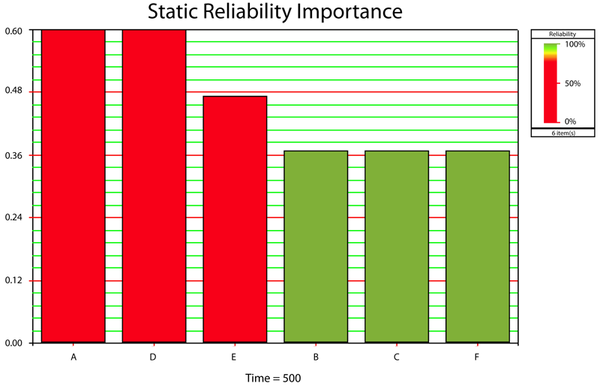# Reliability Importance Example

This example appears in the article Reliability Importance.

Reliability Importance Measures for Failure Modes

Assume that a system has failure modes [math]A\,\![/math], [math]B\,\![/math], [math]C\,\![/math], [math]D\,\![/math], [math]E\,\![/math] and [math]F\,\![/math]. Furthermore, assume that failure of the entire system will occur if:

• Mode [math]B\,\![/math], [math]C\,\![/math] or [math]F\,\![/math] occurs.
• Modes [math]A\,\![/math] and [math]E\,\![/math], [math]A\,\![/math] and [math]D\,\![/math] or [math]E\,\![/math] and [math]D\,\![/math] occur.

In addition, assume the following failure probabilities for each mode.

• Modes [math]A\,\![/math] and [math]D\,\![/math] have a mean time to occurrence of 1,000 hours (i.e., exponential with [math]MTTF=1,000).\,\![/math]
• Mode [math]E\,\![/math] has a mean time to occurrence of 100 hours (i.e., exponential with [math]MTTF=100).\,\![/math]
• Modes [math]B\,\![/math], [math]C\,\![/math] and [math]F\,\![/math] have a mean time to occurrence of 700,000, 1,000,000 and 2,000,000 hours respectively (i.e., exponential with [math]MTT{{F}_{B}}=700,000\,\![/math], [math]MTT{{F}_{C}}=1,000,000\,\![/math] and [math]MTT{{F}_{F}}=2,000,000).\,\![/math]

Examine the mode importance for operating times of 100 and 500 hours.

Solution

The RBD for this example is shown next:

The first chart below illustrates [math]{{I}_{{{R}_{i}}}}(t=100)\,\![/math]. It can be seen that even though [math]B\,\![/math], [math]C\,\![/math] and [math]F\,\![/math] have a much rarer rate of occurrence, they are much more significant at 100 hours. By 500 hours, [math]{{I}_{{{R}_{i}}}}(t=500)\,\![/math], the effects of the lower reliability components become greatly pronounced and thus they become more important, as can be seen in the second chart. Finally, the behavior of [math]{{I}_{{{R}_{i}}}}(t)\,\![/math] can be observed in the Reliability Importance vs. Time plot. Note that not all lines are plainly visible in the plot due to overlap.